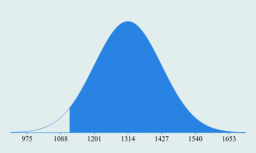# Three sigma rule

Stoach weights are normally distributed, with mean 1314g and standard deviation 113g.

State the probability that a randomly selected stoach weighs more than 1118g.

(Report the probabilities using at least 4 decimal places. )

p =  0.9586

### Step-by-step explanation:Did you find an error or inaccuracy? Feel free to write us. Thank you!

Showing 1 comment:

Tips for related online calculators
Looking for help with calculating arithmetic mean?
Looking for a statistical calculator?
Looking for a standard deviation calculator?

#### Grade of the word problem:

We encourage you to watch this tutorial video on this math problem: